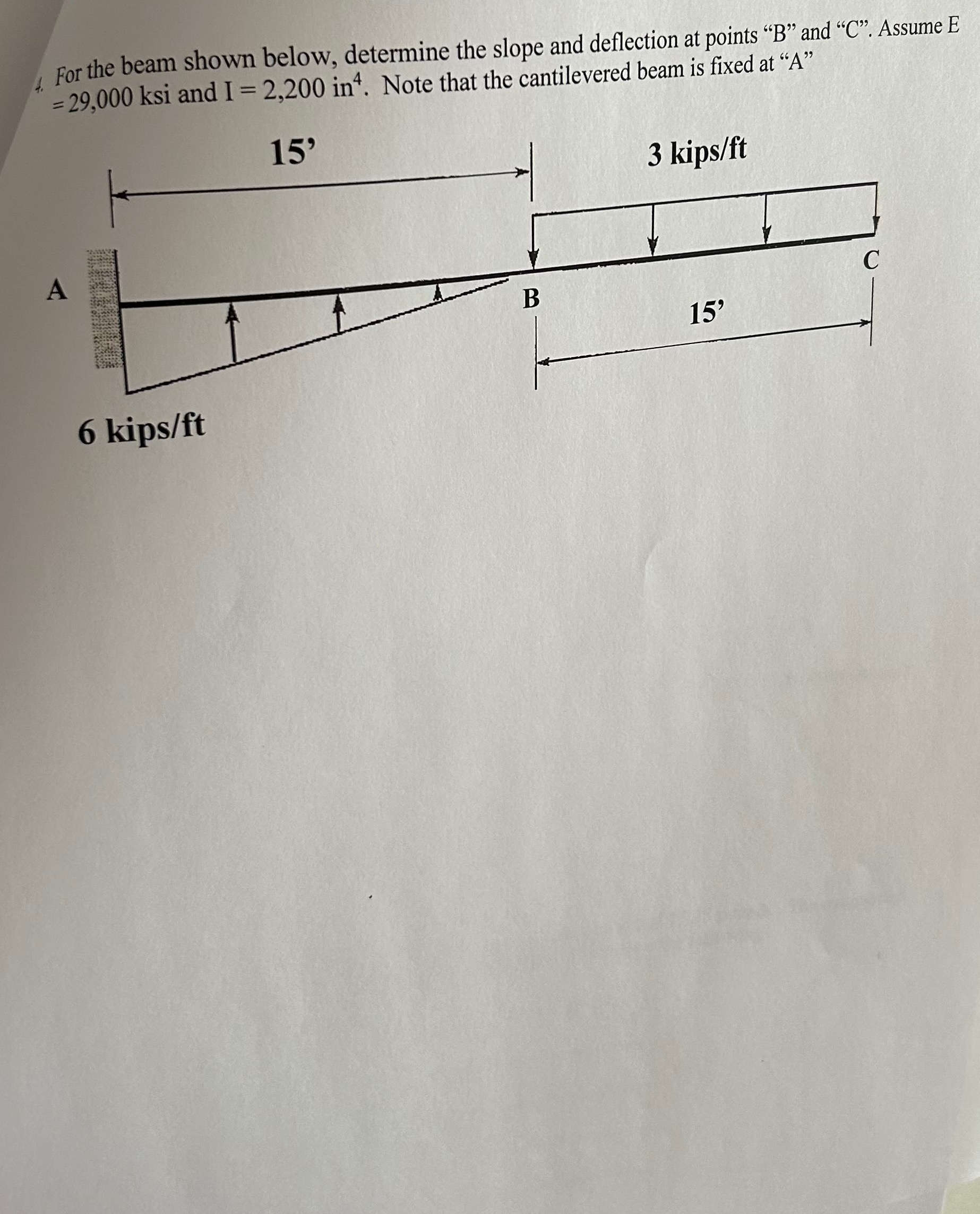Home / Expert Answers / Civil Engineering / for-the-beam-shown-below-determine-the-slope-and-deflection-at-points-34-b-34-and-34-c-34-assume-e-2-pa546

# (Solved): For the beam shown below, determine the slope and deflection at points " B " and " C ". Assume E =2 ...For the beam shown below, determine the slope and deflection at points " " and " ". Assume and . Note that the cantilevered beam is fixed at "A"

We have an Answer from Expert